Mathematics

# x intercept and y intercept

13th Oct '202 views1 mins read

 1 Introduction 2 y intercept 3 How to find y intercept? 4 x intercept 5 How to find x intercept? 6 Find x and y intercepts 7 Summary 8 FAQs

13 October 2020

## Introduction

### Intercept

An intercept is a point where the line or curve passes through the axis of the graph. When a point crosses x axis then that point is called x intercept or horizontal intercept.

And when that point crosses the y axis that point is called y intercept or vertical intercept.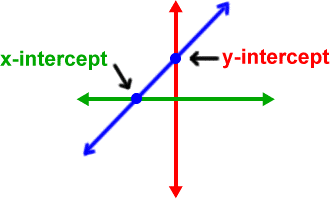Now let us be familiar with the terms used to find the horizontal intercept and vertical intercept.

First is we should know what is the equation of the line

We can write the equation of a straight line as $$y=mx+b$$

Where $$m$$ is called the slope of the line and b is the y intercept.

Now we should know what is meant by the slope of a line

The slope of a line is defined by the slant or steepness of a line.

It is the ratio of change in the y value over change in x value.

It is called “rise over run

$Slope=\frac{\text{change in y value}}{\text{change in x value}}=\frac{\rm{rise}}{\rm{run}}$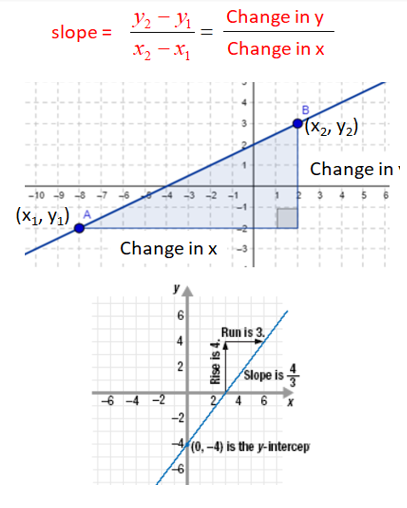Above in the graph, we can find the rise in the graph by taking any two points, it is 4

The run is 3 so the slope here is 4/3.

The slopes are of the following types:-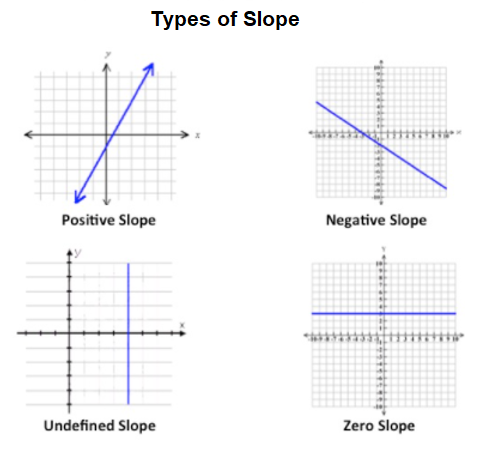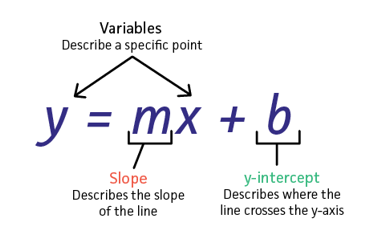Now let us come to y intercept.

## Y intercept

Y intercept is the y value of the point where the line intersects the y axis. We can find the y intercept by looking at the graph and seeing the point which cuts the y axis. The x coordinate of this point will always be zero. It will be written as (0,y). This is also known as vertical intercept since it is situated on the vertical axis.

## How to find y intercept?

To find the y intercept of an equation let x=0 then solve it for y.

## X intercept

### What is the x intercept?

X intercept is the point where a graph of a function or an equation touches the x axis. We can think of this as a point with value 0. This is also called the horizontal intercept since it is situated on the horizontal axis.

## How to find x intercept?

To find the x intercept of an equation let y=0, then solve for x. The co-ordinates for x intercept will be(x,0)

## Find x and y intercepts

Let us do an example.

 Example 1

Find x and y intercepts for the line 6x+3y=18.

How to find x intercept?

set y=0

\begin{align}6x+3(0)&=18\\6x&=18\\x&=\frac{18}{6}\\x&=3\end{align}

 The x intercept is (3,0)

How to find vertical intercept?

set x=0

\begin{align}6(0)+3y&=18\\3y&=18\\y&=\frac{18}{3}\\y&=6\end{align}

 The y intercept is(0,6)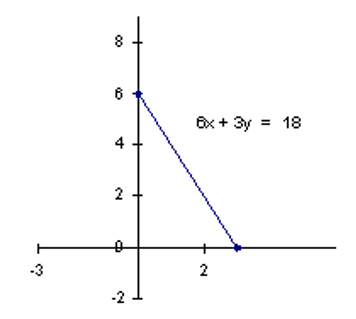### Find x and y intercepts by the graph

Now, x and y intercepts can be found out by looking at the graphs also.

Try to observe the given below graph and find x and y intercepts.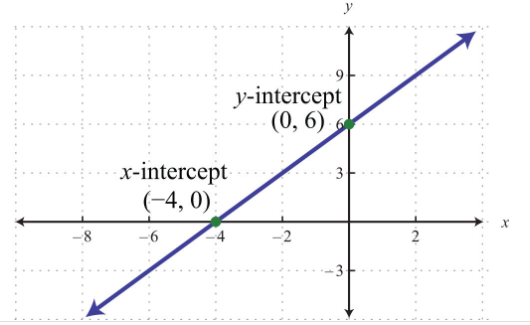How to find the x intercept?

Here, the x intercept is where the line is crossing the x axis. It is at -4 ,so x intercept is (-4,0)

Vertical Intercept

Now the y intercept is the point where it is crossing the y axis.

It is crossing the y axis at 6 so y intercept is(0,6)

### Graphing the line by computing the x and y intercepts when the equation is given.

We can also graph lines by computing the intercepts. Let us see this concept using an example.

 Example 1

Draw the graph of 5x+3y=15 by finding the x and y intercepts.

How to find x intercept?

x intercept is by setting y=0

\begin{align}5x = 15\\x=\frac{15}{5}\\x&=3\end{align}

 X intercept is (3,0)

Vertical Intercept

Y intercept is by setting x=0 in the equation

\begin{align}5(0)+3y&=15\\3y&=15\\y&=5\end{align}

 So y intercept is(0,5)

Plot the x and y intercepts then draw a line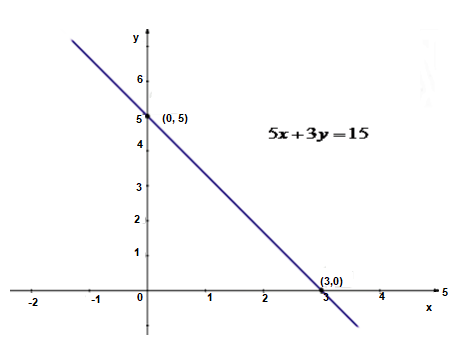### Cases where there are no x intercept and y intercepts

Now keep in mind that the x and y intercepts are not numbers but ordered pairs. Suppose the value of x intercept is 2 it is written as (2,0). In addition, not all graphs have both horizontal and vertical intercepts. For example: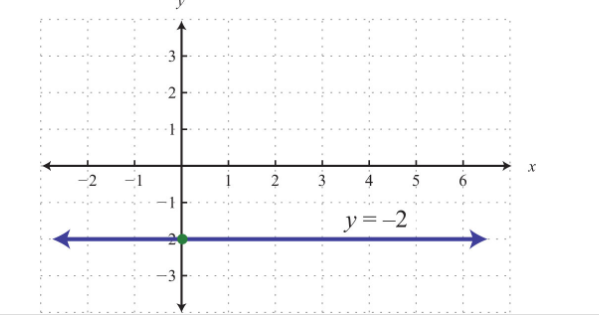The horizontal line graphed above does not have an x intercept. It only has a y intercept as (0,-2).

Now let us see a case where there is no y intercept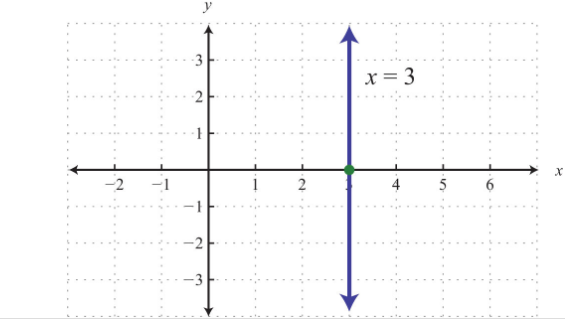The vertical line graphed above has an x intercept (3,0) and no y intercept.

Now, a case where x intercept and y intercept are the same points i.e.origin

Graph after finding x and y intercepts: y=-2x.

To find x intercept x=0

Y=-2(0)

Y=0

So y intercept is (0,0)

To find x intercept put y=0

0= -2x

X=0

So x intercept is(0,0)

So the x intercept and y intercept are actually the same points, the origin. Now we need one more point to graph the line chose any value for x and determine the value for 5

If we take x as 1, we get y=-2

If we take x as -1 we get y=2

Now use these ordered pairs to graph the line(0,0),(-1,2)(1,-2) to graph the line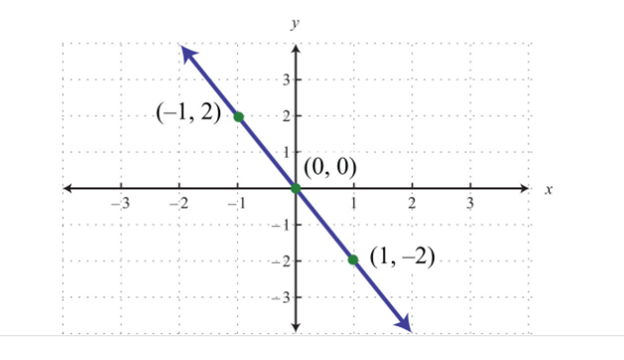## Summary

• The concept of x and y intercept is quite simple.
• The x intercept is the point where the graph cuts the x axis and the y intercept is the point where the graph cuts the y axis.
• So at x intercept, y is 0, And at y intercept, x is 0.
• In the above, we discussed a line whose general equation is:-
• Y= mx+b
• Where m is the slope and b the y intercept
• To find x and y intercept, when the equation is given, set the value of x and y as 0 respectively.
• There is a case where there is no x intercept the graph is a horizontal line and the case where there is no y intercept the graph is a horizontal line.

Note: We discussed here the intercepts related to lines mostly. The intercepts of other functions would be discussed under their own blog.

Written by Nitisha Sahay

## How to find the x intercept?

To find the x intercept of an equation let y=0, then solve for x. The co-ordinates for x intercept will be(x,0).

## What is the x intercept?

X intercept is the point where a graph of a function or an equation touches the x axis. We can think of this as a point with value 0. This is also called the horizontal intercept since it is situated on the horizontal axis.

## How to find y intercept?

To find the y intercept of an equation let x=0 then solve it for y.

Related Articles
GIVE YOUR CHILD THE CUEMATH EDGE
Access Personalised Math learning through interactive worksheets, gamified concepts and grade-wise courses